Question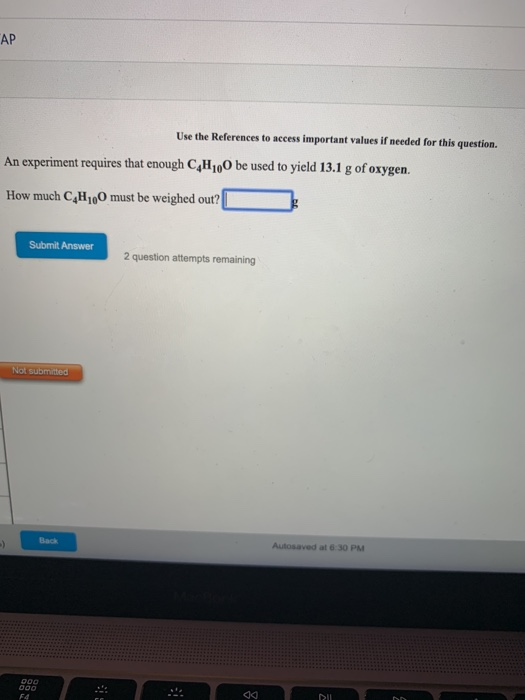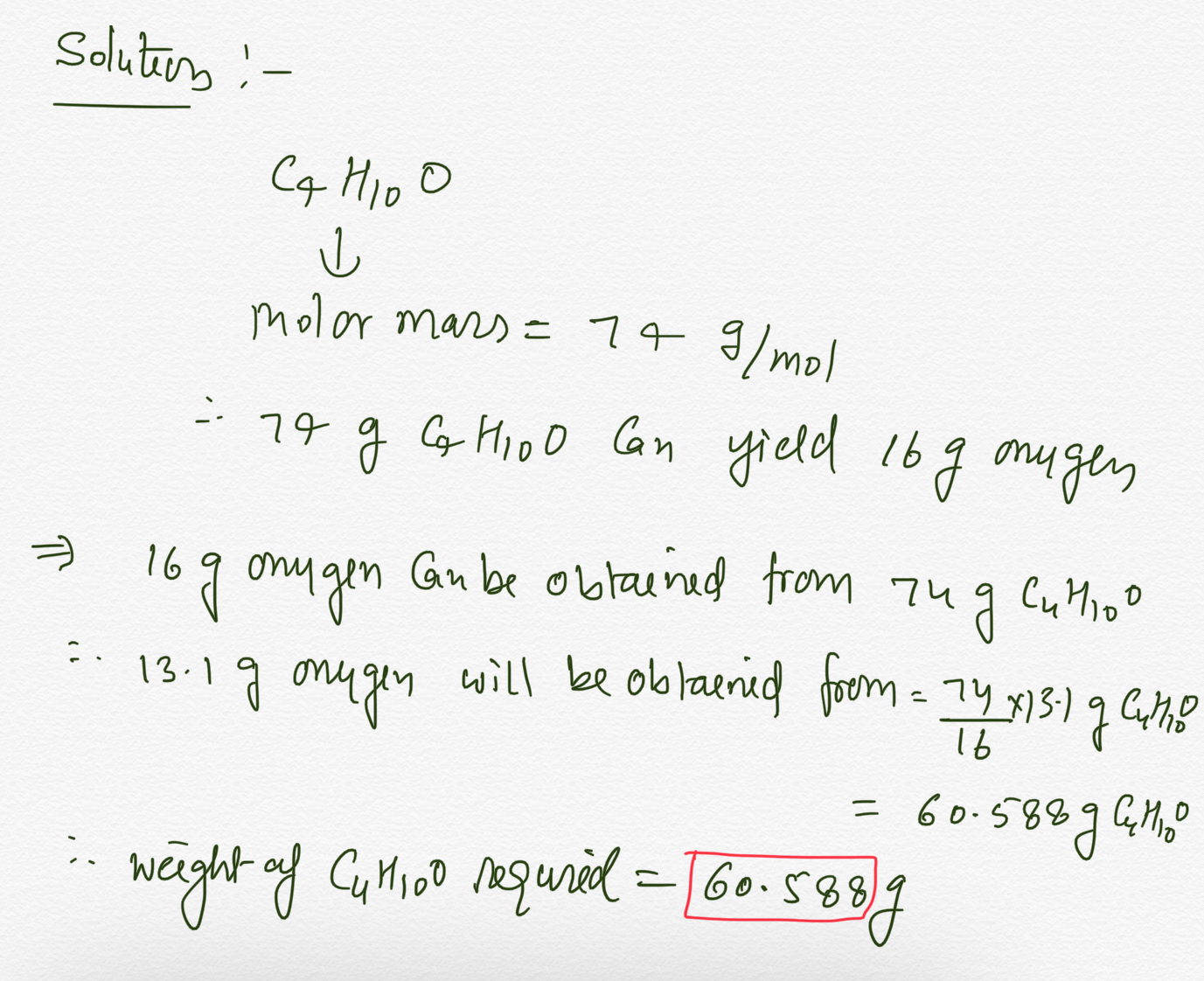#### Earn Coins

Coins can be redeemed for fabulous gifts.

Similar Homework Help Questions
• ### Use the References to access important va An experiment requires that enough B.H,Cl be used to...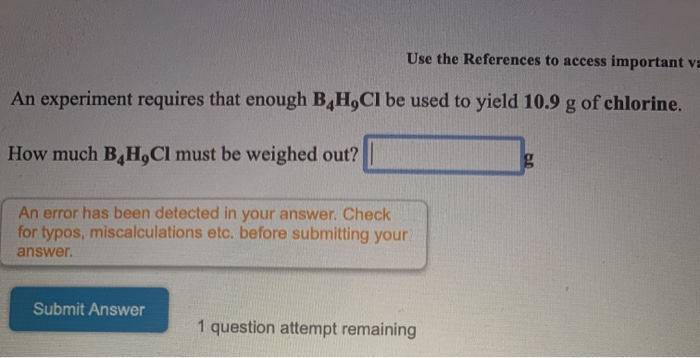Use the References to access important va An experiment requires that enough B.H,Cl be used to yield 10.9 g of chlorine. How much B H,Cl must be weighed out? An error has been detected in your answer. Check! for typos, miscalculations etc. before submitting your answer. Submit Answer 1 question attempt remaining

• ### Use the References to access important values if needed for this question. What volume of oxygen gas is produced w...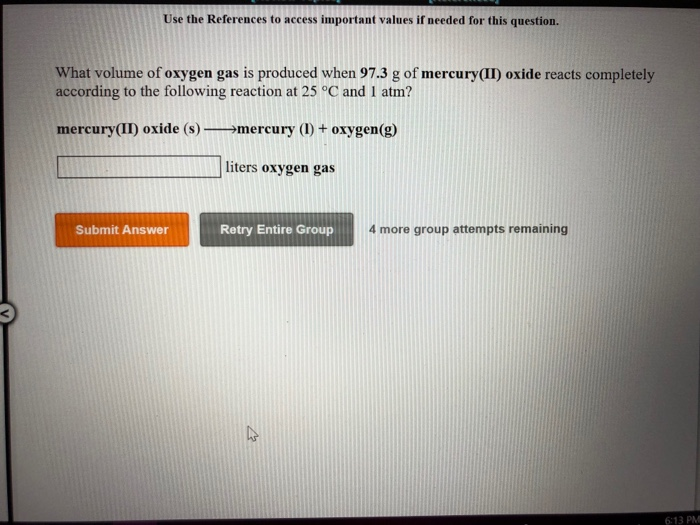Use the References to access important values if needed for this question. What volume of oxygen gas is produced when 97.3 g of mercury(II) oxide reacts completely according to the following reaction at 25 °C and 1 atm? mercury(II) oxide (s) -mercury (1) + oxygen(g) liters oxygen gas 4 more group attempts remaining Submit Answer Retry Entire Group 6:13 PM Review Topics [References] Use the References to access important values if needed for this question. How many grams of carbon...

• ### Use the References to access important values if needed for this question. How many moles of...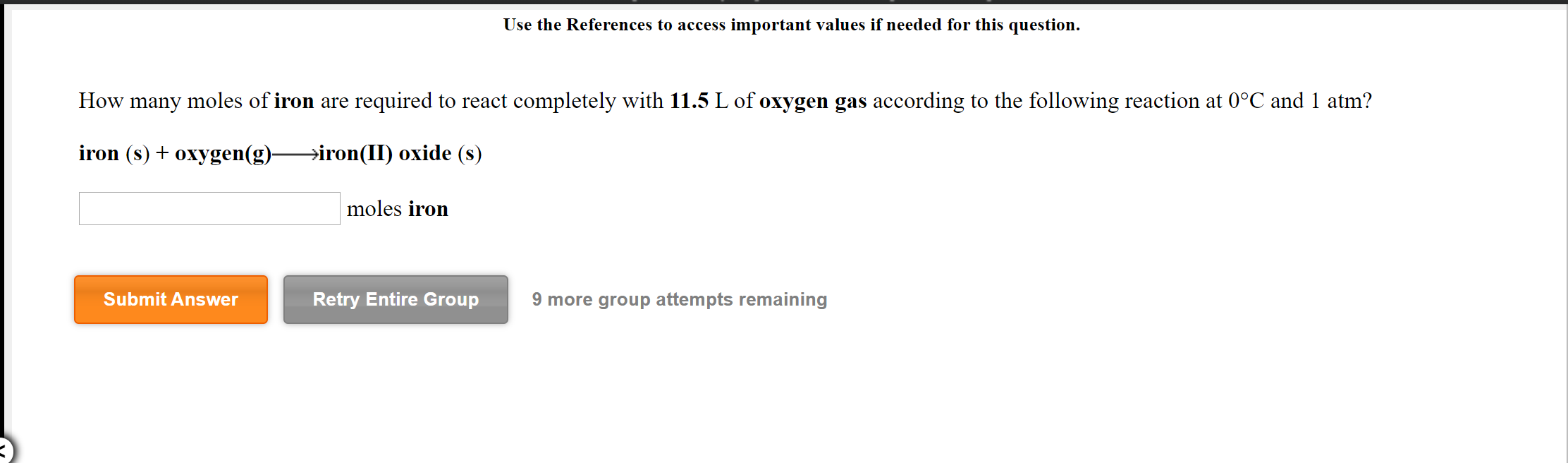Use the References to access important values if needed for this question. How many moles of iron are required to react completely with 11.5 L of oxygen gas according to the following reaction at 0°C and 1 atm? iron (s) + oxygen(g)—>iron(II) oxide (8) moles iron Submit Answer Retry Entire Group 9 more group attempts remaining Use the References to access important values if needed for this question. What volume of oxygen gas is produced when 5.01 mol of water...

• ### RCICICLES Use the References to access important values if needed for this question. What volume of...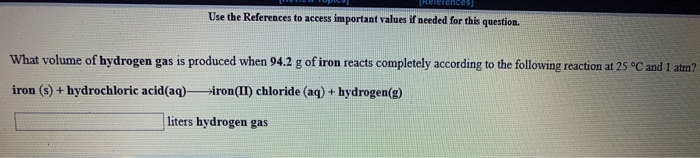RCICICLES Use the References to access important values if needed for this question. What volume of hydrogen gas is produced when 94.2 g of iron reacts completely according to the following reaction at 25 °C and 1 at iron (s) + hydrochloric acid(aq) iron(II) chloride (aq) + hydrogen(s) liters hydrogen gas ILULUI-assignment-take [Review Topics] (References] Use the References to access important values if needed for this question. An iron nail rusts when exposed to oxygen. How many grams of iron...

• ### Use the References to access important values if needed for this question. The pH of an...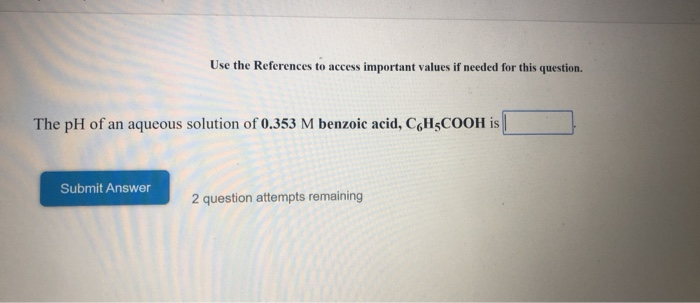Use the References to access important values if needed for this question. The pH of an aqueous solution of 0.353 M benzoic acid, CH5COOH is Submit Answer 2 question attempts remaining Use the References to access important values if needed for this question. The pH of an aqueous solution of 0.353 M hydroxylamine (a weak base with the formula NH OH) is Submit Answer 2 question attempts remaining

• ### Use the References to access important values if needed for this question. You need to make...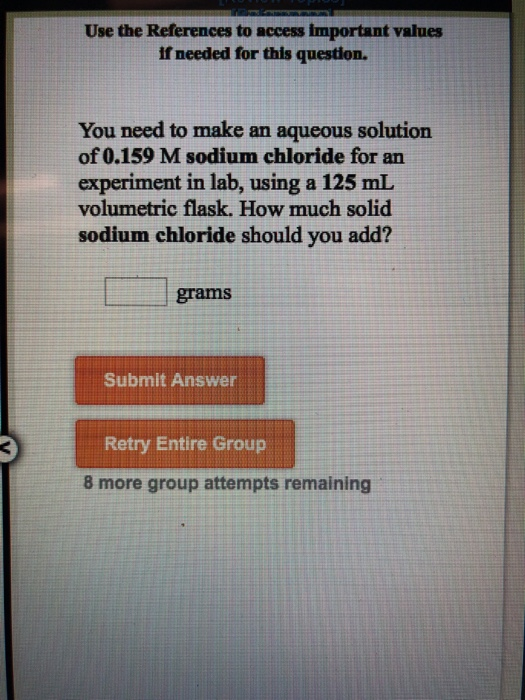Use the References to access important values if needed for this question. You need to make an aqueous solution of 0.159 M sodium chloride for an experiment in lab, using a 125 ml volumetric flask. How much solid sodium chloride should you add? grams Submit Answer Retry Entire Group 8 more group attempts remaining Use the References to access important values if needed for this question. In the laboratory you dissolve 14.7 g of potassium sulfate in a volumetric flask...

• ### Use the References to access important values if needed for this question. What volume of propane...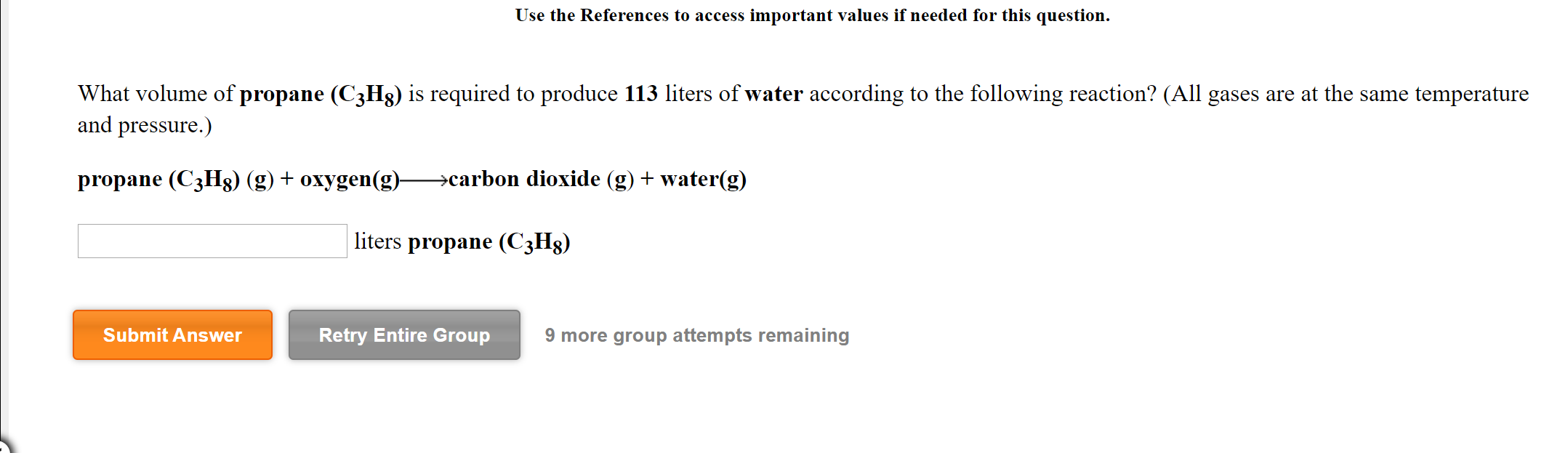Use the References to access important values if needed for this question. What volume of propane (C3H8) is required to produce 113 liters of water according to the following reaction? (All gases are at the same temperature and pressure.) propane (C3Hg) (g) + oxygen(g)—>carbon dioxide (g) + water(g) liters propane (C3H2) Submit Answer Retry Entire Group 9 more group attempts remaining Use the References to access important values if needed for this question. What volume of carbon dioxide is produced...

• ### Review Topics) References Use the References to access important values if needed for this question A...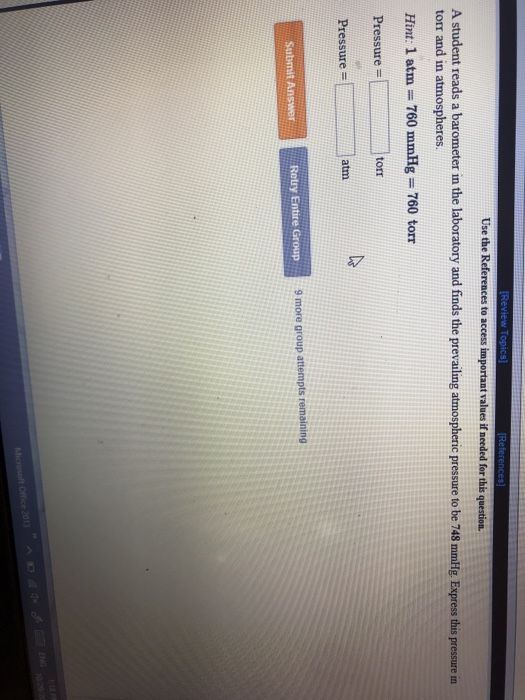Review Topics) References Use the References to access important values if needed for this question A student reads a barometer in the laboratory and finds the prevailing atmospheric pressure to be 748 mmHg. Express this pressure in torr and in atmospheres. Hint: 1 atm 760 mmHg 760 torr Pressure = torr Pressure = atm Submit Answer Retry Entire Group 9 more group attempts remaining ENG 10292 Micreseft Office 2013 Review Topics Use the References to access important values if needed...

• ### References Use the References to access important values if needed for this question. For the following...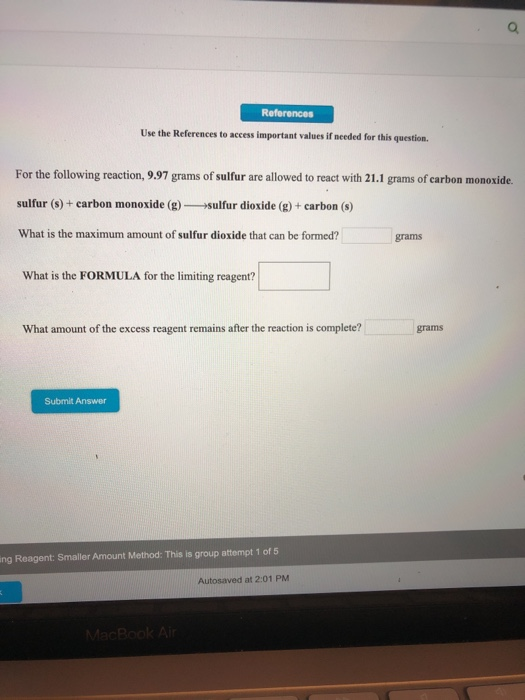References Use the References to access important values if needed for this question. For the following reaction, 9.97 grams of sulfur are allowed to react with 21.1 grams of carbon monoxide. sulfur () + carbon monoxide (g) sulfur dioxide (g) + carbon (8) What is the maximum amount of sulfur dioxide that can be formed? grams What is the FORMULA for the limiting reagent? What amount of the excess reagent remains after the reaction is complete? grams Submit Answer ing...

• ### Use the References to access important values if needed for this question. A mixture of krypton...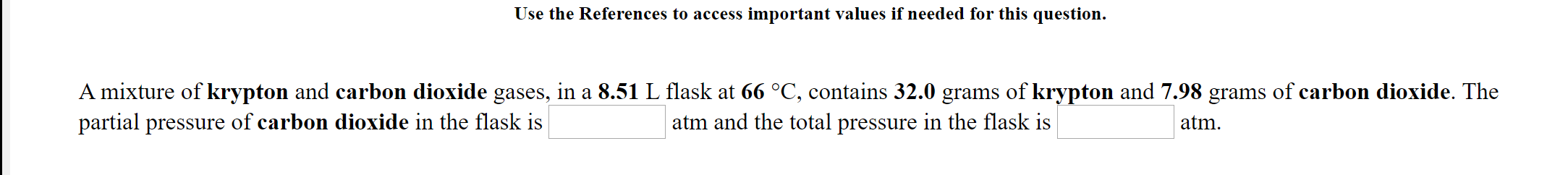Use the References to access important values if needed for this question. A mixture of krypton and carbon dioxide gases, in a 8.51 L flask at 66 °C, contains 32.0 grams of krypton and 7.98 grams of carbon dioxide. The partial pressure of carbon dioxide in the flask is atm and the total pressure in the flask is atm. Use the References to access important values if needed for this question. A mixture of oxygen and neon gases is maintained...